SLIS135E December   2010  – February 2017

PRODUCTION DATA.

1. Features
2. Applications
3. Description
4. Revision History
5. Pin Configuration and Functions
6. Specifications
7. Detailed Description
1. 7.1 Overview
2. 7.2 Functional Block Diagram
3. 7.3 Feature Description
4. 7.4 Device Functional Modes
5. 7.5 Programming
6. 7.6 Register Map
8. Application and Implementation
1. 8.1 Application Information
2. 8.2 Typical Application
9. Power Supply Recommendations
10. 10Layout
11. 11Device and Documentation Support
12. 12Mechanical, Packaging, and Orderable Information

• RTE|16
• RTE|16

## 6 Specifications

### 6.1 Absolute Maximum Ratings

over operating free-air temperature range (unless otherwise noted)(1) (2) (3)
MIN MAX UNIT
Supply voltage VDD to GND –0.3 7 V
All other pins to GND –0.3 VDD + 0.3 V
IL
IW
IH
Pulse current ±20 mA
Continuous current TPL0202-10 ±2 mA
Tstg Storage temperature –65 150 °C
Stresses beyond those listed under Absolute Maximum Ratings may cause permanent damage to the device. These are stress ratings only, which do not imply functional operation of the device at these or any other conditions beyond those indicated under Recommended Operating Conditions. Exposure to absolute-maximum-rated conditions for extended periods may affect device reliability.
Follows the algebraic convention, whereby the most negative value is a minimum and the most positive value is a maximum.
All voltages are with respect to ground, unless otherwise specified.

### 6.2 ESD Ratings

VALUE UNIT
V(ESD) Electrostatic discharge Human-body model (HBM), per ANSI/ESDA/JEDEC JS-001(1) ±2500 V
Charged-device model (CDM), per JEDEC specification JESD22-C101(2) ±1000
JEDEC document JEP155 states that 500-V HBM allows safe manufacturing with a standard ESD control process.
JEDEC document JEP157 states that 250-V CDM allows safe manufacturing with a standard ESD control process.

### 6.3 Recommended Operating Conditions

over operating free-air temperature range (unless otherwise noted)
MIN MAX UNIT
VDD,GND 2.7 5.5 V
VH, VL, VW Terminal voltage range 0 VDD V
VIH Voltage input high (SCLK, DIN, CS) VDD = 3.6 V to 5.5 V 2.4 5.5 V
VDD = 2.7 V to 3.6 V 0.7 × VDD 5.5
VIL Voltage input low (SCLK, DIN, CS) 0 0.8 V
IW Wiper current ±2 mA
TA Free-air ambient temperature –40 105 °C

### 6.4 Thermal Information

THERMAL METRIC(1) TPL0202 UNIT
RTE (WQFN)
16 PINS
RθJA Junction-to-ambient thermal resistance 73.2 °C/W
RθJC(top) Junction-to-case (top) thermal resistance 33.4 °C/W
RθJB Junction-to-board thermal resistance 34.9 °C/W
ψJT Junction-to-top characterization parameter 0.3 °C/W
ψJB Junction-to-board characterization parameter 34.9 °C/W
RθJC(bot) Junction-to-case (bottom) thermal resistance 23.5 °C/W
For more information about traditional and new thermal metrics, see the Semiconductor and IC Package Thermal Metrics application report.

### 6.5 Electrical Characteristics

VDD = 2.7 to 5.5 V, TA= –40°C to +105°C (unless otherwise noted). Typical values are at VDD= 5 V, TA= 25°C (unless otherwise noted).
PARAMETER TEST CONDITIONS MIN TYP MAX UNIT
RTOT End-to-end resistance
(between H and L terminals)
VL = VDD / 2, IHL = 100 µA, Input code = 0x80, Measure VHL 8 10 12
RH High terminal resistance VL = VDD / 2, IHL = 100 µA, Input code = 0xFF, Measure VHW 100 200 Ω
RL Low terminal resistance VL = VDD / 2, IHL = 100 µA, Input code = 0x00, Measure VWL 60 200 Ω
RW Wiper resistance VL = VDD / 2, IWL = 100 µA, Input code = 0x00, Measure VHW 25 100 Ω
CH, CL (14) (15) Terminal capacitance 22 pF
CW (14) (15) Wiper capacitance 18 pF
ILKG Terminal leakage current VH = VSS to VDD, VL = Floating
OR
VL = VSS to VDD, VH = Floating
0.1 1 µA
TCR Resistance temperature coefficient Input code = 0x80h 132 ppm/°C
RTOT,MATCH Channel-to-channel resistance match 0.1%
VOLTAGE DIVIDER MODE
INL(1) (3) Integral non-linearity –1 1 LSB
DNL(1) (4) Differential non-linearity –0.5 0.5 LSB
ZSERROR (2) (5) Zero-scale error 0 2 5 LSB
FSERROR (2) (6) Full-scale error –5 –2 0 LSB
VMATCH (2) (7) Channel-to-channel matching Wiper at the same tap position, same voltage all H and the same voltage at all L terminals –2 2 LSB
TCV Ratiometric temperature coefficient Wiper set at midscale 12 ppm/°C
BW Bandwidth Wiper set at midscale
CLOAD = 10 pF
VL = VDD / 2,
Signal applied to H; measurement at W
2000 kHz
two Register write to output time Time from CS rising edge to 90% of expected value 2 µs
THD+N Total harmonic distortion + noise VHL = 1 VRMS at 1 kHz,
VL = VDD / 2,
Measurement at W
0.03%
XTALK Crosstalk fH_A = 1 kHz,
VL_A = VL_B = VDD / 2, VH_B = Floating
Measurement at W_A and W_B
–94 dB
RHEOSTAT MODE (Measurements between W and L with H not connected, or between W and H with L not connected)
RINL (8) (10) Integral non-linearity –1.5 1.5 LSB
RDNL(8) (11) Differential non-linearity –0.5 0.5 LSB
ROFFSET (9) (12) Offset 0 2.5 7 LSB
RMATCH (9) (13) Channel-to-channel matching –2 2 LSB
LSB = (VMEAS[code 255] – VMEAS[code 0]) / 255
IDEAL_LSB = (VH – VL) / 256
INL = ((VMEAS[code x] – VMEAS[code 0]) / LSB) – [code x]
DNL = ((VMEAS[code x] – VMEAS[code x-1]) / LSB) – 1
ZSERROR = VMEAS[code 0] / IDEAL_LSB
FSERROR = [(VMEAS[code 255] – (VH – VL)) / IDEAL_LSB] + 1
VMATCH = (VMEAS_A[code x] – VMEAS_B[code x]) / IDEAL_LSB
RLSB = (RMEAS[code 255] – RMEAS[code 0]) / 255
IDEAL_RLSB = RTOT / 256
RINL =((RMEAS[code x] – RMEAS[code 0]) / RLSB) - [code x]
RDNL = ((RMEAS[code x] – RMEAS[code x-1]) / RLSB ) – 1
ROFFSET = RMEAS[code 0] / IDEAL_RLSB
RMATCH = (RMEAS_A[code x] – RMEAS_B[code x]) / IDEAL_RLSB
Terminal and wiper capacitance extracted from self admittance of three-port network measurement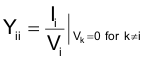Digital potentiometer macromodel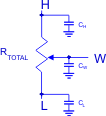### 6.6 Operating Characteristics

VDD = 2.7 V to 5.5 V, VH= VDD, VL= GND, TA= –40°C to +105°C (unless otherwise noted). Typical values are at VDD= 5 V, TA= 25°C (unless otherwise noted).
PARAMETER TEST CONDITIONS MIN TYP MAX UNIT
IDD(STBY) VDD supply current during standby Digital inputs = VDD or GND 1 5 µA
IDD VDD supply current during write cycle only Digital inputs = VDD or GND 400 µA
IIN-DIG Digital pins leakage current (SCLK, DIN, CS inputs) –1 1 µA
VPOR Power-on recall voltage Minimum VDD at which memory recall occurs 2 V
EEPROM SPECIFICATION
EEPROM write endurance TA = 105°C 1000 cycles
TA = 25°C 10000
EEPROM retention TA = 105°C 20 years
TA = 85°C 100
tBUSY Write NV register busy time 20 ms
tACC Read NV register access time Time from CS rising edge to wiper start to 10% of expected change with read NVM command 40 ns
tD Power-up response time (VDD above VPOR to wiper register value recall completed) Time from VPOR to wiper output settled 35 100 µs
SERIAL INTERFACE SPECIFICATIONS (SCLK, DIN, CS INPUTS)
VIH Input high voltage VDD = 3.6 to 5.5 V 2.4 5.5 V
VDD = 2.7 to 3.6 V 0.7 × VDD 5.5
VIL Input low voltage SCLK, DIN, CS inputs 0 0.8 V
CIN Pin capacitance SCLK, DIN, CS inputs 7 pF

### 6.7 SPI Timing Requirements

VDD = 2.7 V to 5.5 V, VH= VDD, VL= GND, TA= –40°C to +105°C (unless otherwise noted)
MIN MAX UNIT
fSCLK SCLK frequency 5 MHz
tSCP SCLK period 200 ns
tSCH SCLK high time 80 ns
tSCL SCLK low time 80 ns
tCSS CS fall to SCLK rise setup time 80 ns
tCSH SCLK rise to CS hold time 0 ns
tDS DIN to SCLK setup time 50 ns
tDH DIN hold after SCLK rise to CS fall 0 ns
tCS0 SCLK rise to CS fall 20 ns
tCS1 CS rise to SCLK rise hold 80 ns
tCSW CS pulse width high 200 ns

### 6.8 Typical Characteristics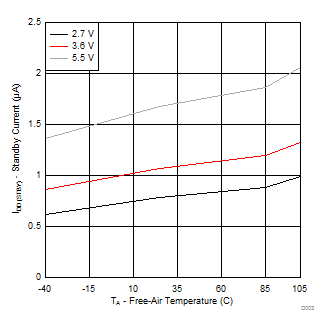Figure 1. Standby Current vs Temperature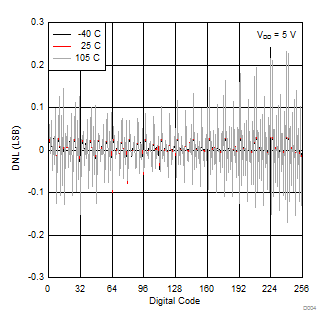Figure 3. Voltage Divider Mode DNL vs Temperature
(VDD = 5 V)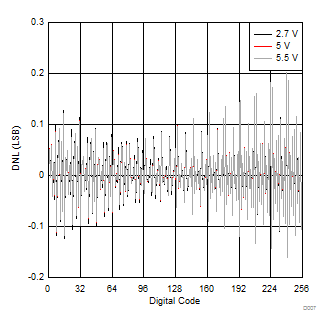Figure 5. Voltage Divider Mode DNL vs Supply Voltage (25°C)Figure 7. Voltage Divider Mode Unadjusted Error (VDD = 5V)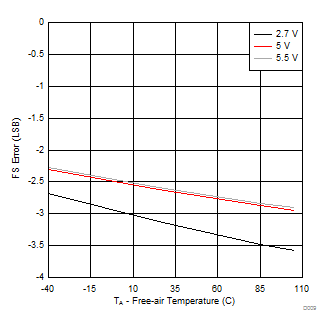Figure 9. Voltage Divider Mode FS Error vs Temperature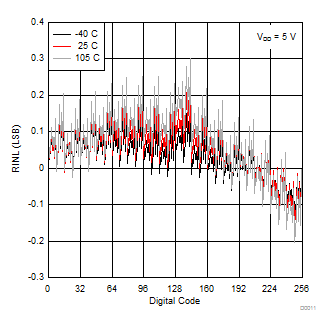Figure 11. Rheostat Mode RINL vs Temperature (VDD = 5 V)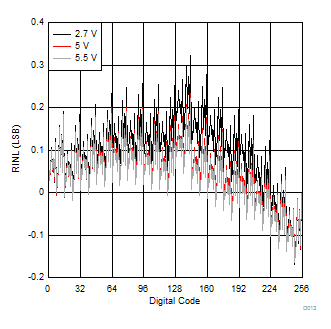Figure 13. Rheostat Mode RINL vs Supply Voltage (25°C)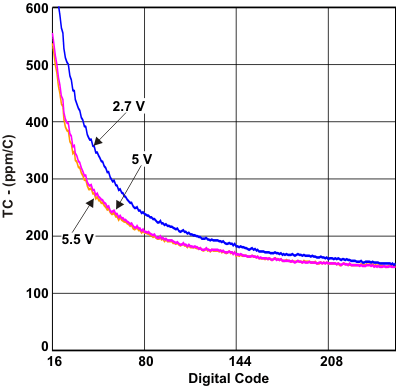Figure 15. Rheostat Mode TC vs Digital Code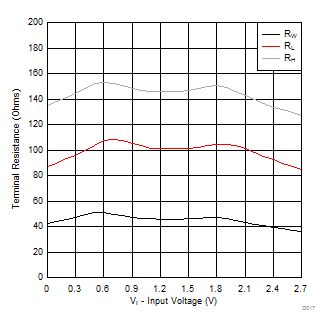Figure 17. Wiper and Terminal Resistance (VDD = 2.7 V)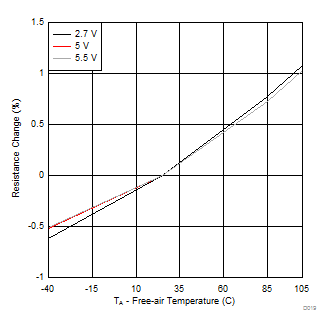Figure 19. End-End Resistance Change vs Temperature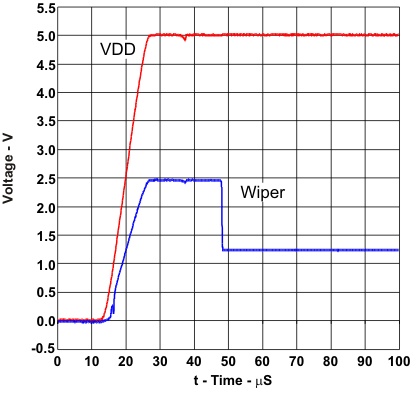Figure 21. tPOR (Power-Up Response Time) Non-Volatile Memory = 40h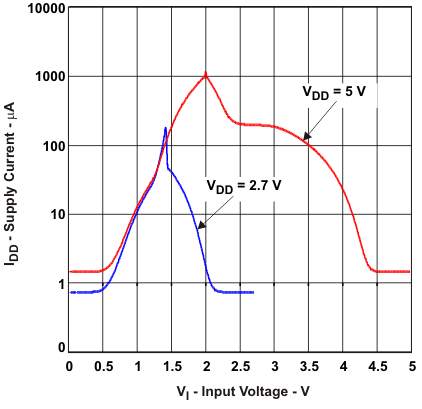Figure 2. Supply Current vs Digital Input Voltage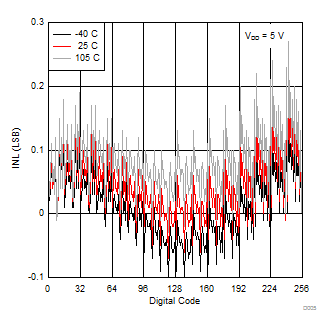Figure 4. Voltage Divider Mode INL vs Temperature
(VDD = 5 V)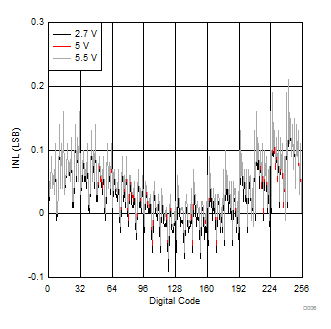Figure 6. Voltage Divider Mode INL vs Supply Voltage (25°C)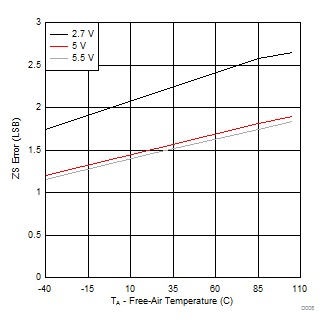Figure 8. Voltage Divider Mode ZS Error vs Temperature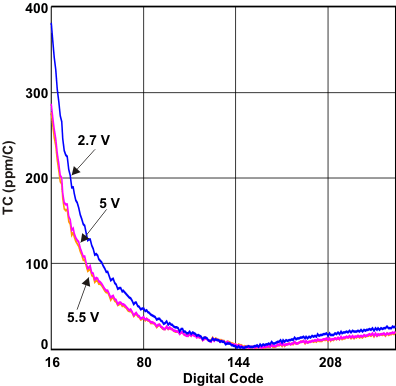Figure 10. Voltage Divider Mode vs Digital Code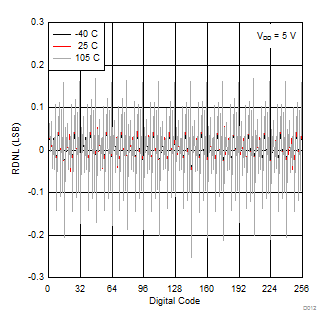Figure 12. Rheostat Mode RDNL vs Temperature (VDD = 5 V)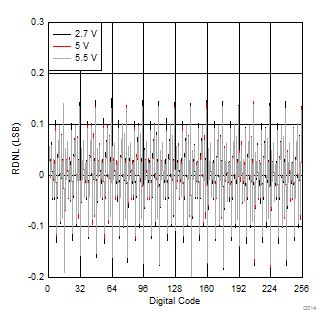Figure 14. Rheostat Mode RDNL vs Supply Voltage (25°C)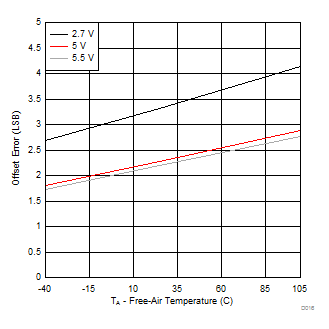Figure 16. Rheostat Mode Offset Error vs TemperatureFigure 18. Wiper and Terminal Resistance (VDD = 5 V)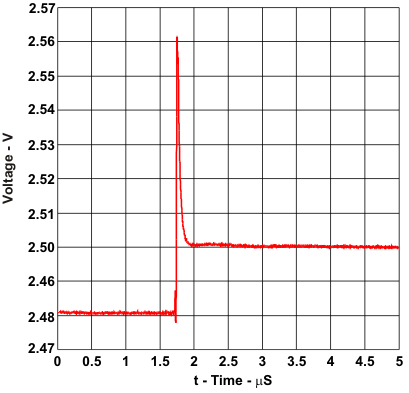Figure 20. Midscale Wiper Glitch (Code 7fh to 80h) VDD = 5 V, VH = VDD, VL = GND, Cload = 10 pF